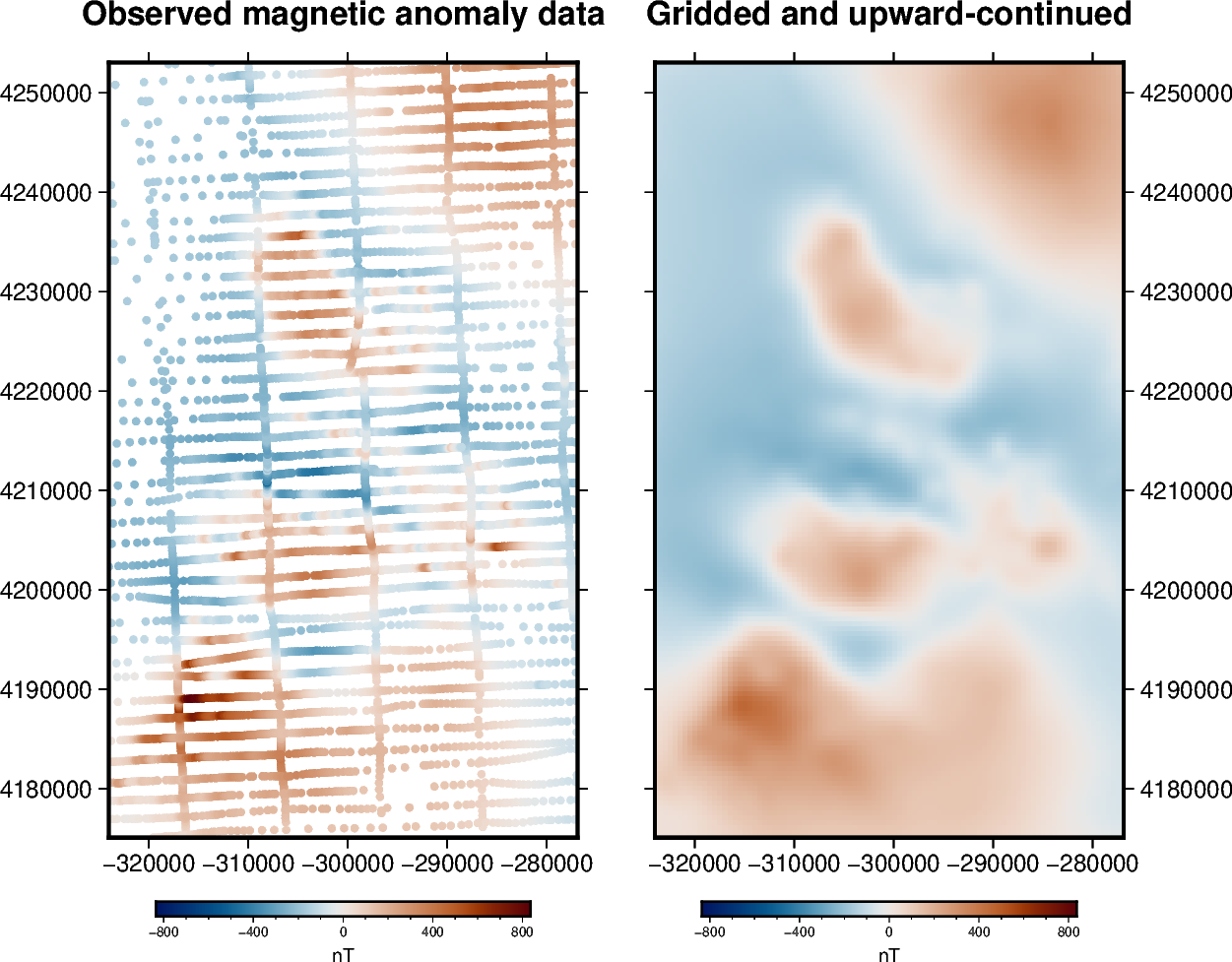# Gridding with block-averaged equivalent sources¶

By default, the `harmonica.EquivalentSources` class locates one point source beneath each data point during the fitting process. Alternatively, we can use another strategy: the block-averaged sources, introduced in [Soler2021].

This method divides the survey region (defined by the data) into square blocks of equal size, computes the median coordinates of the data points that fall inside each block and locates one source beneath every averaged position. This way, we define one equivalent source per block, with the exception of empty blocks that won’t get any source.

This method has two main benefits:

• It lowers the amount of sources involved in the interpolation, therefore it reduces the computer memory requirements and the computation time of the fitting and prediction processes.

• It might avoid to produce aliasing on the output grids, specially for surveys with oversampling along a particular direction, like airborne ones.

We can make use of the block-averaged sources within the `harmonica.EquivalentSources` class by passing a value to the `block_size` parameter, which controls the size of the blocks. We recommend using a `block_size` not larger than the desired resolution of the interpolation grid.

The depth of the sources can be set analogously to the regular equivalent sources: we can use a `constant` depth (every source is located at the same depth) or a `relative` depth (where each source is located at a constant shift beneath the median location obtained during the block-averaging process). The depth of the sources and which strategy to use can be set up through the `depth` and the `depth_type` parameters, respectively.Out:

```Number of data points: 7054
Mean height of observations: 541.8293166997448
/usr/share/miniconda3/envs/testing/lib/python3.9/site-packages/sklearn/linear_model/_base.py:148: FutureWarning: 'normalize' was deprecated in version 1.0 and will be removed in 1.2. Please leave the normalize parameter to its default value to silence this warning. The default behavior of this estimator is to not do any normalization. If normalization is needed please use sklearn.preprocessing.StandardScaler instead.
warnings.warn(
R² score: 0.9955764795499585

Generated grid:
<xarray.Dataset>
Dimensions:           (northing: 157, easting: 95)
Coordinates:
* easting           (easting) float64 -3.24e+05 -3.235e+05 ... -2.769e+05
* northing          (northing) float64 4.175e+06 4.176e+06 ... 4.253e+06
upward            (northing, easting) float64 1.5e+03 1.5e+03 ... 1.5e+03
Data variables:
magnetic_anomaly  (northing, easting) float64 28.63 28.56 ... 168.6 158.7
Attributes:
metadata:  Generated by EquivalentSources(block_size=500, damping=1, dept...
```

```import matplotlib.pyplot as plt
import pyproj
import verde as vd

import harmonica as hm

# Fetch the sample total-field magnetic anomaly data from Great Britain
data = hm.datasets.fetch_britain_magnetic()

# Slice a smaller portion of the survey data to speed-up calculations for this
# example
region = [-5.5, -4.7, 57.8, 58.5]
inside = vd.inside((data.longitude, data.latitude), region)
data = data[inside]
print("Number of data points:", data.shape)
print("Mean height of observations:", data.altitude_m.mean())

# Since this is a small area, we'll project our data and use Cartesian
# coordinates
projection = pyproj.Proj(proj="merc", lat_ts=data.latitude.mean())
easting, northing = projection(data.longitude.values, data.latitude.values)
coordinates = (easting, northing, data.altitude_m)

# Create the equivalent sources.
# We'll use block-averaged sources at a constant depth beneath the observation
# points. We will interpolate on a grid with a resolution of 500m, so we will
# use blocks of the same size. The damping parameter helps smooth the predicted
# data and ensure stability.
eqs = hm.EquivalentSources(depth=1000, damping=1, block_size=500, depth_type="constant")

# Fit the sources coefficients to the observed magnetic anomaly.
eqs.fit(coordinates, data.total_field_anomaly_nt)

# Evaluate the data fit by calculating an R² score against the observed data.
# This is a measure of how well the sources fit the data, NOT how good the
# interpolation will be.
print("R² score:", eqs.score(coordinates, data.total_field_anomaly_nt))

# Interpolate data on a regular grid with 500 m spacing. The interpolation
# requires the height of the grid points (upward coordinate). By passing in
# 1500 m, we're effectively upward-continuing the data (mean flight height is
# 500 m).
grid = eqs.grid(upward=1500, spacing=500, data_names=["magnetic_anomaly"])

# The grid is a xarray.Dataset with values, coordinates, and metadata
print("\nGenerated grid:\n", grid)

# Plot original magnetic anomaly and the gridded and upward-continued version
fig, (ax1, ax2) = plt.subplots(nrows=1, ncols=2, figsize=(12, 9), sharey=True)

# Get the maximum absolute value between the original and gridded data so we
# can use the same color scale for both plots and have 0 centered at the white
# color.
maxabs = vd.maxabs(data.total_field_anomaly_nt, grid.magnetic_anomaly.values)

ax1.set_title("Observed magnetic anomaly data")
tmp = ax1.scatter(
easting,
northing,
c=data.total_field_anomaly_nt,
s=20,
vmin=-maxabs,
vmax=maxabs,
cmap="seismic",
)
plt.colorbar(tmp, ax=ax1, label="nT", pad=0.05, aspect=40, orientation="horizontal")
ax1.set_xlim(easting.min(), easting.max())
ax1.set_ylim(northing.min(), northing.max())

ax2.set_title("Gridded and upward-continued")
tmp = grid.magnetic_anomaly.plot.pcolormesh(
ax=ax2,
vmin=-maxabs,
vmax=maxabs,
cmap="seismic",
)
plt.colorbar(tmp, ax=ax2, label="nT", pad=0.05, aspect=40, orientation="horizontal")
ax2.set_xlim(easting.min(), easting.max())
ax2.set_ylim(northing.min(), northing.max())

plt.show()
```

Total running time of the script: ( 0 minutes 2.579 seconds)

Gallery generated by Sphinx-Gallery